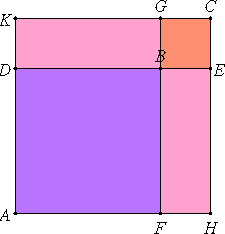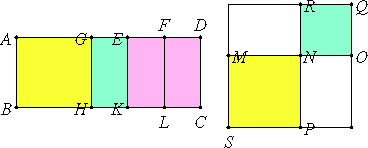# Lemma

Let there be two squares AB and BC, and let them be placed so that DB is in a straight line with BE. Then FB is also in a straight line with BG. Complete the parallelogram AC.

I say that AC is a square, that DG is a mean proportional between AB and BC, and further that DC is a mean proportional between AC and CB.

Since DB equals BF, and BE is to BG, therefore the whole DE equals the whole FG.I.34

But DE equals each of the straight lines AH and KC, and FG equals each of the straight lines AK and HC, therefore each of the straight lines AH and KC also equals each of the straight lines AK and HC.

Therefore the parallelogram AC is equilateral. And it is also rectangular, therefore AC is a square.

Since FB is to BG as DB is to BE, while FB is to BG as AB is to DG, and DB is to BE as DG is to BC, therefore AB is to DG as DG is to BC.

Therefore DG is a mean proportional between AB and BC.

I say next that DC is also a mean proportional between AC and CB.

Since AD is to DK as KG is to GC, for they are equal respectively, and, taken jointly, AK is to KD as KC is to CG, while AK is to KD as AC is to CD, and AC is to CG as DC is to CB, therefore AC is to DC as DC is to BC.

Therefore DC is a mean proportional between AC and CB.

Q.E.D.

# Proposition 54

If an area is contained by a rational straight line and the first binomial, then the side of the area is the irrational straight line which is called binomial.

Let the area AC be contained by the rational straight line AB and the first binomial AD.

I say that the side of the area AC is the irrational straight line which is called binomial.

Since AD is a first binomial straight line, divide it into its terms at E, and let AE be the greater term.X.Def.II.1

It is then manifest that AE and ED are rational straight lines commensurable in square only, the square on AE is greater than the square on ED by the square on a straight line commensurable with AE, and AE is commensurable in length with the rational straight line AB set out.

Bisect ED at the point F.

X.17

Then, since the square on AE is greater than the square on ED by the square on a straight line commensurable with AE, therefore, if there is applied to the greater AE a parallelogram equal to the fourth part of the square on the less, that is, to the square on EF, and deficient by a square figure, then it divides it into commensurable parts.

Apply to AE the rectangle AG by GE equal to the square on EF. Then AG is commensurable in length with EG.

Draw GH, EK, and FL from G, E, and F parallel to either of the straight lines AB and CD. Construct the square SN equal to the parallelogram AH, and the square NQ equal to GK, and place them so that MN is in a straight line with NO. Then RN is also in a straight line with NP. Complete the parallelogram SQ. Then SQ is a square.

VI.17

Now, since the rectangle AG by GE equals the square on EF, therefore AG is to EF as FE is to EG.

VI.1

Therefore AH is to EL as EL is to KG. Therefore EL is a mean proportional between AH and GK.

Lemma

But AH equals SN, and GK equals NQ, therefore EL is a mean proportional between SN and NQ. But MR is also a mean proportional between the same SN and NQ, therefore EL equals MR, so that it also equals PO.

But AH and GK also equal SN and NQ, therefore the whole AC equals the whole SQ, that is, it equals the square on MO, Therefore MO is the side of AC.

I say next that MO is binomial.

X.15

Since AG is commensurable with GE, therefore AE is also commensurable with each of the straight lines AG and GE.

X.12

But AE is also, by hypothesis, commensurable with AB, therefore AG and GE are also commensurable with AB.

X.19

And AB is rational, therefore each of the straight lines AG and GE is also rational. Therefore each of the rectangles AH and GK is rational, and AH is commensurable with GK.

But AH equals SN, and GK equals NQ, therefore the sum of SN and NQ, that is the squares on MN and NO, are rational and commensurable.

Since AE is incommensurable in length with ED, while AE is commensurable with AG, and DE is commensurable with EF, therefore AG is also incommensurable with EF, so that AH is also incommensurable with EL.

But AH equals SN, and EL equals MR, therefore SN is also incommensurable with MR. But SN is to MR as PN is to NR, therefore PN is incommensurable with NR.

But PN equals MN, and NR equals NO, therefore MN is incommensurable with NO. And the square on MN is commensurable with the square on NO, and each is rational, therefore MN and NO are rational straight lines commensurable in square only.

X.36

Therefore MO is binomial and the side of AC.

Therefore, if an area is contained by a rational straight line and the first binomial, then the side of the area is the irrational straight line which is called binomial.

Q.E.D.

## Guide

The lemma before the proposition is used in this proposition, X.60, and X.11. The proposition itself is used in X.71.